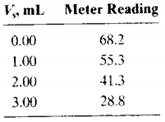### Create an Account

Home / Questions / The following volumes of a standard 100 ppb F solution were added to four 1000mLaliquots o...

# The following volumes of a standard 100 ppb F solution were added to four 1000mLaliquots of a water sample

The following volumes of a standard 10.0 ppb F solution were added to four 10.00-mLaliquots of a water sample: 0.00, 1.00, 2.00, and 3.00 mL. Precisely 5.00 mL of a solution containing an excess of the strongly absorbing AI-acid alizarin garnet R complex was added to each of the four solutions, and they were each diluted to 50.0 mL. The fluorescence intensities of the four solutions were as follows:(a) Explain the chemistry of the analytical method.

(b) Construct a plot of the data.

(c) Use the fact that the fluorescence decreases with increasing amounts of the F- standard to derive a relationship like Equation 1-3 for multiple standard additions. Use that relationship further to obtain an equation for the unknown concentration cin terms of the slope and intercept of the standard additions plot, similar to Equcation 1-4.

(d) Use linear least squares to find the equation for the line representing the decrease in fluorescence relative to the volume of standard fluoride Vs.

(e) Calculate the standard deviation of the slope and intercept.

(f) Calculate the concentration of F- in the sample in parts per billion.

(g) Calculate the standard deviation of the result in (e).

Jun 22 2020 View more View LessSubscribe To Get Solution